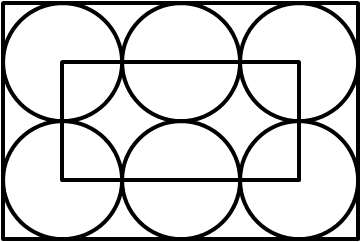#### You may also like### Hallway Borders

What are the possible dimensions of a rectangular hallway if the number of tiles around the perimeter is exactly half the total number of tiles?### Square Pegs

Which is a better fit, a square peg in a round hole or a round peg in a square hole?### Boxed In

A box has faces with areas 3, 12 and 25 square centimetres. What is the volume of the box?

# Six Circles

##### Age 11 to 14 ShortChallenge LevelIn the diagram, six circles of equal size touch adjacent circles and the sides of the large rectangle.

Each of the corners of the small rectangle is the centre of one of the large circles.

The perimeter of the small rectangle is $60\text{cm}$.

What is the perimeter of the large rectangle?

If you liked this problem, here is an NRICH task which challenges you to use similar mathematical ideas.

This problem is taken from the UKMT Mathematical Challenges.
You can find more short problems, arranged by curriculum topic, in our short problems collection.